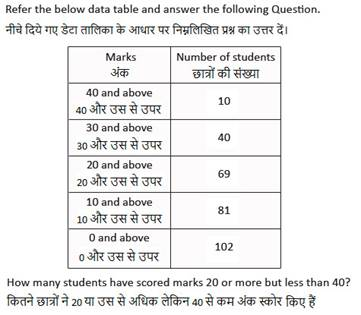# SSC CHSL (Tier - 1) Online Exam Paper - 2016 "held on 20 January 2017" Morning Shift (Quantitative Aptitude)## SSC CHSL (Tier - 1) Online Exam Paper - 2016 "held on 20 January 2017" Morning Shift (Quantitative Aptitude)

QID : 51 - What are the co-ordinates of the centroid of a triangle, whose vertices are A(-2,5), B(3,-2) and C(5,3)?

Options:

1) (-2,-2)

2) (-2,2)

3) (2,2)

4) (2,-2)

Correct Answer: (2,2)

QID : 52 - Ravindra walks at 6 km/hr  and Riya cycles at 11 km/hr  towards each  other. What was the distance between them when they started if they meet  after 42 minutes?

Options:

1) 17.9 kms

2) 14.9 kms

3) 8.9 kms

4) 11.9 kms

Correct Answer: 11.9 kms

QID : 53 - If cot 135° = x, then the value of x is

Options:

1) -1/√3

2) -√3

3) -1

4) -1/2

Correct Answer: -1

QID : 54 - The slopes of two lines are 1/√3  and √3. What is the angle between these two lines?

Options:

1) 15°

2) 45°

3) 60°

4) 30°

Correct Answer: 30°

QID : 55 - If √(1 - cos2A)/cosA = x, then the value of x is

Options:

1) cotA

2) cosecA

3) tanA

4) secA

Correct Answer: tanA

QID : 56 - If x + 2(3-5x) > -1 - 2x < 5 - x/3; then the value of x is

Options:

1) -3

2) -4

3) 2

4) 3

Correct Answer: -3

QID : 57 - An angle is four times its complementary angle. What is the measure of the angle?

Options:

1) 72°

2) 108°

3) 54°

4) 36°

Correct Answer: 72°

QID : 58 - In a Kite  .............

Options:

1) One diagonal bisects the other

2) Both diagonals form two congruent triangles

3) Adjacent  angles are supplementary

4) Opposite sides are parallel

Correct Answer: One diagonal bisects the other

## Online Coaching for SSC CHSL (10+2) Examination

QID : 59 - Akhilesh is two times as good a workman as Bansi and therefore is able to ﬁnish a job in 39 days less  than Bansi. Working together, they can do it in

Options:

1) 13 days

2) 24 days

3) 26 days

4) 12 days

Correct Answer: 26 days

QID : 60 - If (4x - 3) = (3x + 8), then the numerical value of (x + 1)3  is

Options:

1) 1331

2) 1000

3) 1728

4) 729

Correct Answer: 1728

QID : 61 - A rectangular swimming pool of length 30 m and breadth 15 m has  a uniformly sloping  floor. It is 1 m in depth  on one end of its length and 2 m at the other. How much water is needed to completely ﬁll this pool?

Options:

1) 1350 cubic mts

2) 2025 cubic mts

3) 675 cubic mts

4) 2700 cubic mts

Correct Answer: 675 cubic mts

QID : 62 - The sum of all prime numbers between 58 and 62 is

Options:

1) 116

2) 114

3) 120

4) 111

Correct Answer: 120

QID : 63 - 25% discount is offered on an item. By applying a promo code  the customer wins 22% cash back. What is the effective discount?

Options:

1) 41.5 percent

2) 50.63 percent

3) 47 percent

4) 27.5 percent

Correct Answer: 41.5 percent

QID : 64 - 39 is 5.2% of?

Options:

1) 75

2) 750

3) 1500

4) 1875

Correct Answer: 750

QID : 65 - A sum fetched a total simple  interest of Rs. 8100 at the rate of 6% per year in 9 years.  What is the sum?

Options:

1) Rs 15000

2) Rs 18000

3) Rs 12000

4) Rs 9000

Correct Answer: Rs 15000

QID : 66 - If a - b = -6 and a2  + b2  = 44, then ab is

Options:

1) -4

2) 8

3) 4

4) -8

Correct Answer: 4

QID : 67 - The ﬁrst and last terms of an arithmetic progression are 29 and -49. If the sum of the series is -140, then it has  how many terms?

Options:

1) 13

2) 14

3) 12

4) 11

Correct Answer: 14

QID : 68 - The average weight of Sudesh, Jyothi and Ritu is 89 kg. If the average weight of Sudesh and Jyothi be 98 kg and that of Jyothi and Ritu be 83 kg, then the weight of Jyothi is

Options:

1) 58

2) 56

3) 95

4) 66

Correct Answer: 95

QID : 69 - By increasing the price of entry ticket to a fair in the ratio 7:17, the number of visitors to the fair has  decreased in the ratio 9:5. In what ratio has  the total collection increased or decreased?

Options:

1) decreased in the ratio 85:63

2) increased in the ratio 35:153

3) increased in the ratio 63:85

4) decreased in the ratio 153:35

Correct Answer: increased in the ratio 63:85

QID : 70 - What is the value of √[(1 - sinA)/(1  + sinA)]?

Options:

1) cosA/(1 - sinA)

2) cosecA/(1 + sinA)

3) cosA/(1 + sinA)

4) cosecA/(1 - sinA)

Correct Answer: cosA/(1 + sinA)

QID : 71 - Raghuram sells a machine for Rs 43 lakhs at a loss.  Had he sold it for Rs 53 lakh, his gain would have been  7 times the former loss.  Find the cost price of the machine.

Options:

1) Rs 51.75 lakhs

2) Rs 59.14 lakhs

3) Rs 36.75 lakhs

4) Rs 44.25 lakhs

Correct Answer: Rs 44.25 lakhs

QID : 72 -Options:

1) 31 : 35

2) 35 : 31

3) 37 : 33

4) 33 : 37

Correct Answer: 35 : 31

QID : 73 -Options:

1) 69

2) 40

3) 109

4) 59

Correct Answer: 59

QID : 74 -Options:

1) \$1.03 trillion

2) \$1.04 trillion

3) \$0.93 trillion

4) \$1.07 trillion

Correct Answer: \$1.04 trillion

QID : 75 -Options:

1) 37

2) 42

3) 47

4) 52

Correct Answer: 47

## << Go Back to Main Page

IMP! GET SSC EXAM ALERTS on EMAIL